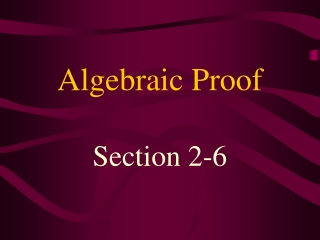DownloadDownload PresentationAlgebraic Proof

# Algebraic Proof

Download Presentation## Algebraic Proof

- - - - - - - - - - - - - - - - - - - - - - - - - - - E N D - - - - - - - - - - - - - - - - - - - - - - - - - - -
##### Presentation Transcript

1. Algebraic Proof Section 2-6

2. Properties of Equality • Addition Property: If a = b and c = d, then a + c = b + d. • Subtraction Property: If a = b and c = d, then a – c = b – d. • Multiplication Property: If a = b, then ca = cb. • Division Property: If a = b and then

3. Substitution Property: If a = b, then either a or b may be substituted for the other in any equation or inequality. • Reflexive Property: a = a • Symmetric Property: If a = b, then b = a • Transitive Property: If a = b and b = c, then a = c.

4. Distributive Property: a(b + c) = ab + ac

5. Properties of Congruence • Reflexive Property: • Symmetric Property: • Transitive Property:

6. Joke Time What do you get if you cross a dentist and a boat? The tooth ferry!

7. What happened to the frog’s car when the parking meter expired? It got toad away!